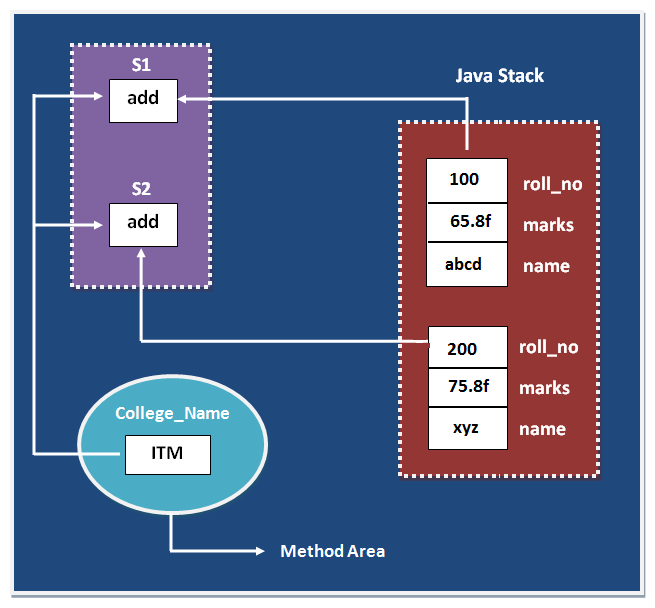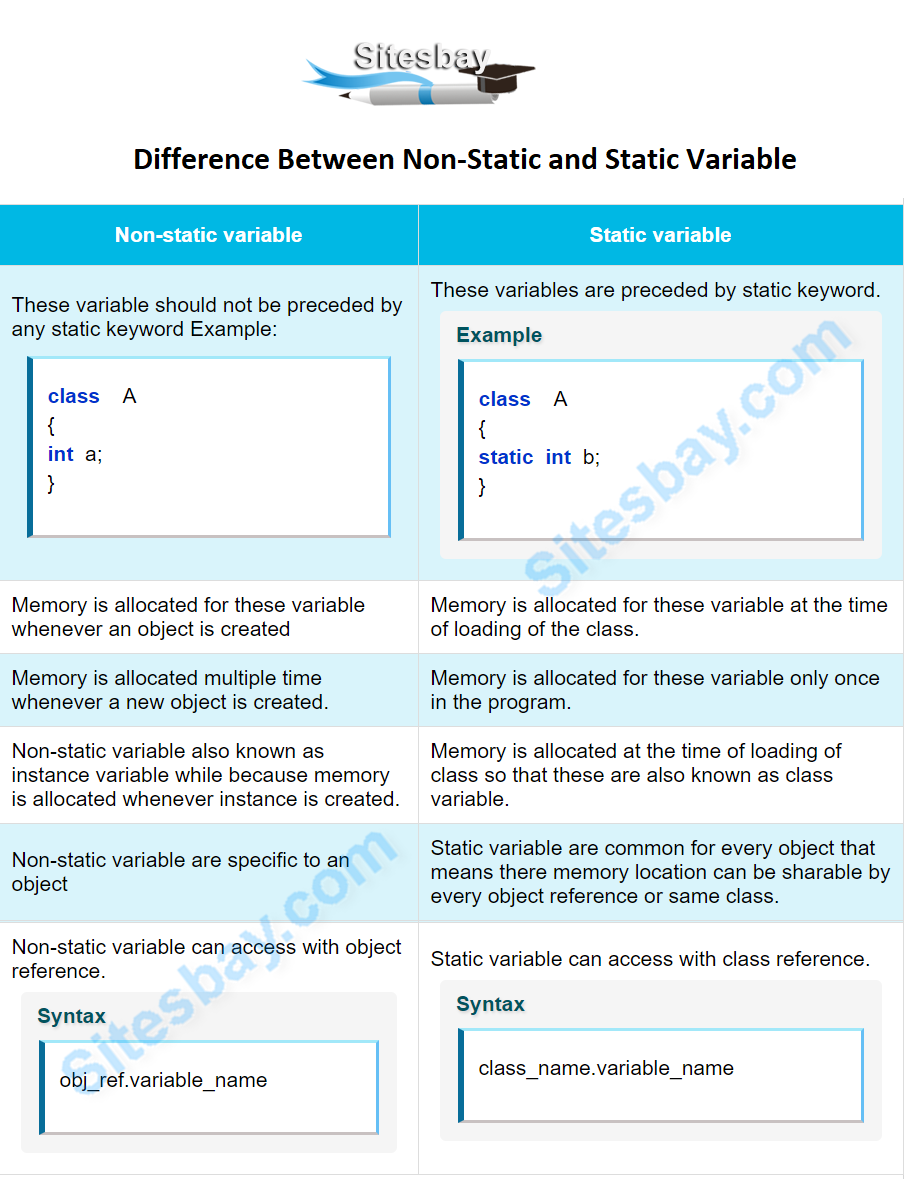# Static and non-static variable in Java

## Difference Between Static and non-Static Variable in Java

The variable of any class are classified into two types;

• Static or class variable
• Non-static or instance variable

### Static variable in Java

Memory for static variable is created only one in the program at the time of loading of class. These variables are preceded by static keyword. tatic variable can access with class reference.

### Non-static variable in Java

Memory for non-static variable is created at the time of create an object of class. These variable should not be preceded by any static keyword Example: These variables can access with object reference.

## Difference between non-static and static variable

Non-static variableStatic variable
1These variable should not be preceded by any static keyword Example:
```class  A
{
int a;
}
```
These variables are preceded by static keyword.

## Example

```class  A
{
static int b;
}
```
2Memory is allocated for these variable whenever an object is createdMemory is allocated for these variable at the time of loading of the class.
3Memory is allocated multiple time whenever a new object is created.Memory is allocated for these variable only once in the program.
4Non-static variable also known as instance variable while because memory is allocated whenever instance is created.Memory is allocated at the time of loading of class so that these are also known as class variable.
5Non-static variable are specific to an objectStatic variable are common for every object that means there memory location can be sharable by every object reference or same class.
6Non-static variable can access with object reference.

## Syntax

```obj_ref.variable_name
```
Static variable can access with class reference.

## Syntax

```class_name.variable_name
```

Note: static variable not only can be access with class reference but also some time it can be accessed with object reference.

## Example

```class Student
{
int roll_no;
float marks;
String name;
static String College_Name="ITM";
}
class StaticDemo
{
public static void main(String args[])
{
Student s1=new Student();
s1.roll_no=100;
s1.marks=65.8f;
s1.name="abcd";
System.out.println(s1.roll_no);
System.out.println(s1.marks);
System.out.println(s1.name);
System.out.println(Student.College_Name);
//or System.out.println(s1. College_Name); but first is use in real time.
Student  s2=new  Student();
s2.roll_no=200;
s2.marks=75.8f;
s2.name="zyx";
System.out.println(s2.roll_no);
System.out.println(s2.marks);
System.out.println(s2.name);
System.out.println(Student.College_Name);
}
}
```

## Output

```100
65.8
abcd
ITM
200
75.8
zyx
ITM
```Note: In the above example College_Name variable is commonly sharable by both S1 and S2 objects.

## Understand static and non-static variable using counter

### Program of counter without static variable

In this example, we have created an instance variable named count which is incremented in the constructor. Since instance variable gets the memory at the time of object creation, each object will have the copy of the instance variable, if it is incremented, it won't reflect to other objects. So each objects will have the value 1 in the count variable.

## Example

```class Counter
{
int count=0;//will get memory when instance is created
Counter()
{
count++;
System.out.println(count);
}
public static void main(String args[])
{
Counter c1=new Counter();
Counter c2=new Counter();
Counter c3=new Counter();
}
}
```

## Output

```1
1
1
```

### Program of counter by static variable

As we have mentioned above, static variable will get the memory only once, if any object changes the value of the static variable, it will retain its value.

## Example

```class Counter
{
static int count=0; //will get memory only once
Counter()
{
count++;
System.out.println(count);
}
public static void main(String args[])
{
Counter c1=new Counter();
Counter c2=new Counter();
Counter c3=new Counter();
}
}
```

## Output

```1
2
3
```WIFI CCTV Camera